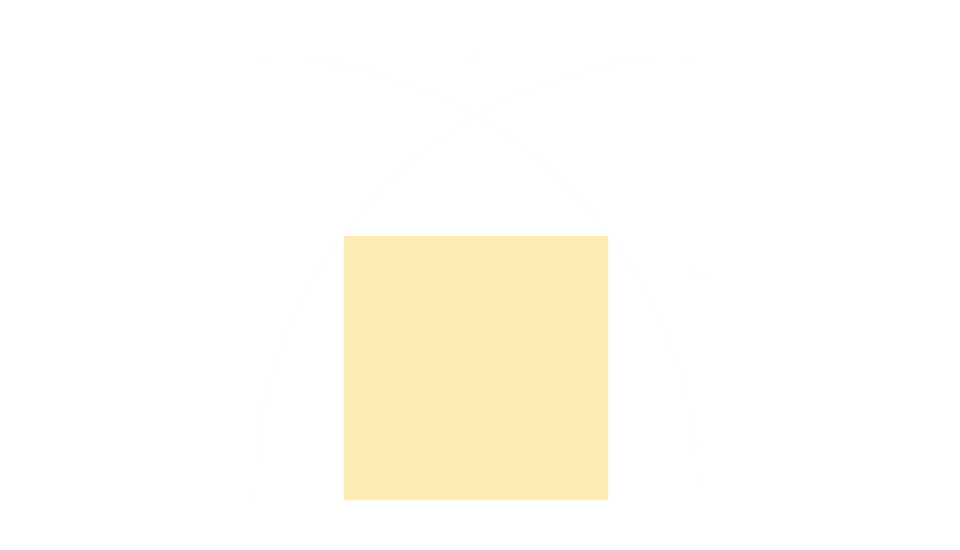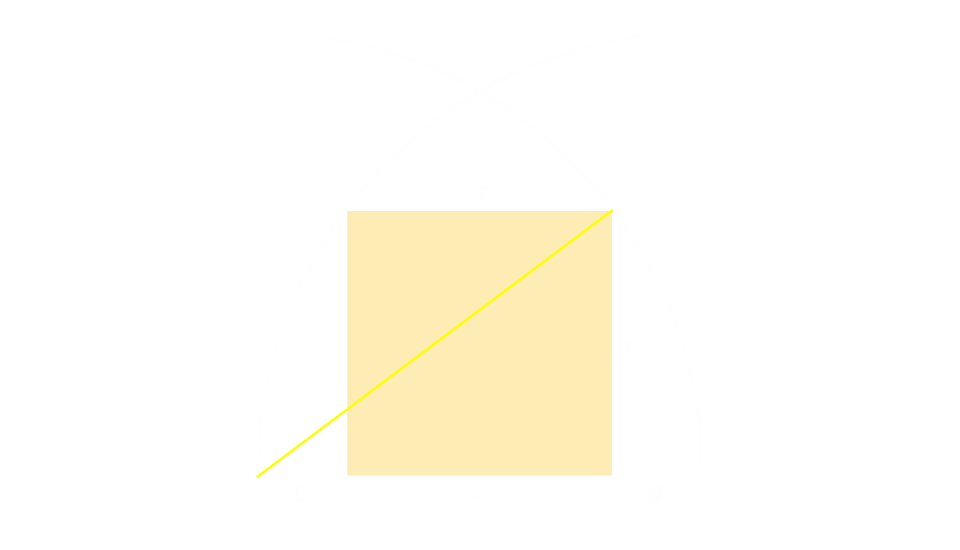# Square and Arc Puzzle

A smaller shaded square is wedged between two quarter circle arcs. What is the ratio of the smaller square to the larger square?What is the ratio of the smaller square to the larger square?

## Solution

We'll define the side of the smaller square as x. Through symmetry, there is an equal gap either side, which we'll call a. The side of the larger square is, therefore (x+2a).The yellow line is the radius of the circle, and is also length (x+2a).
Using Pythagoras, we can derive an equation between x and a: